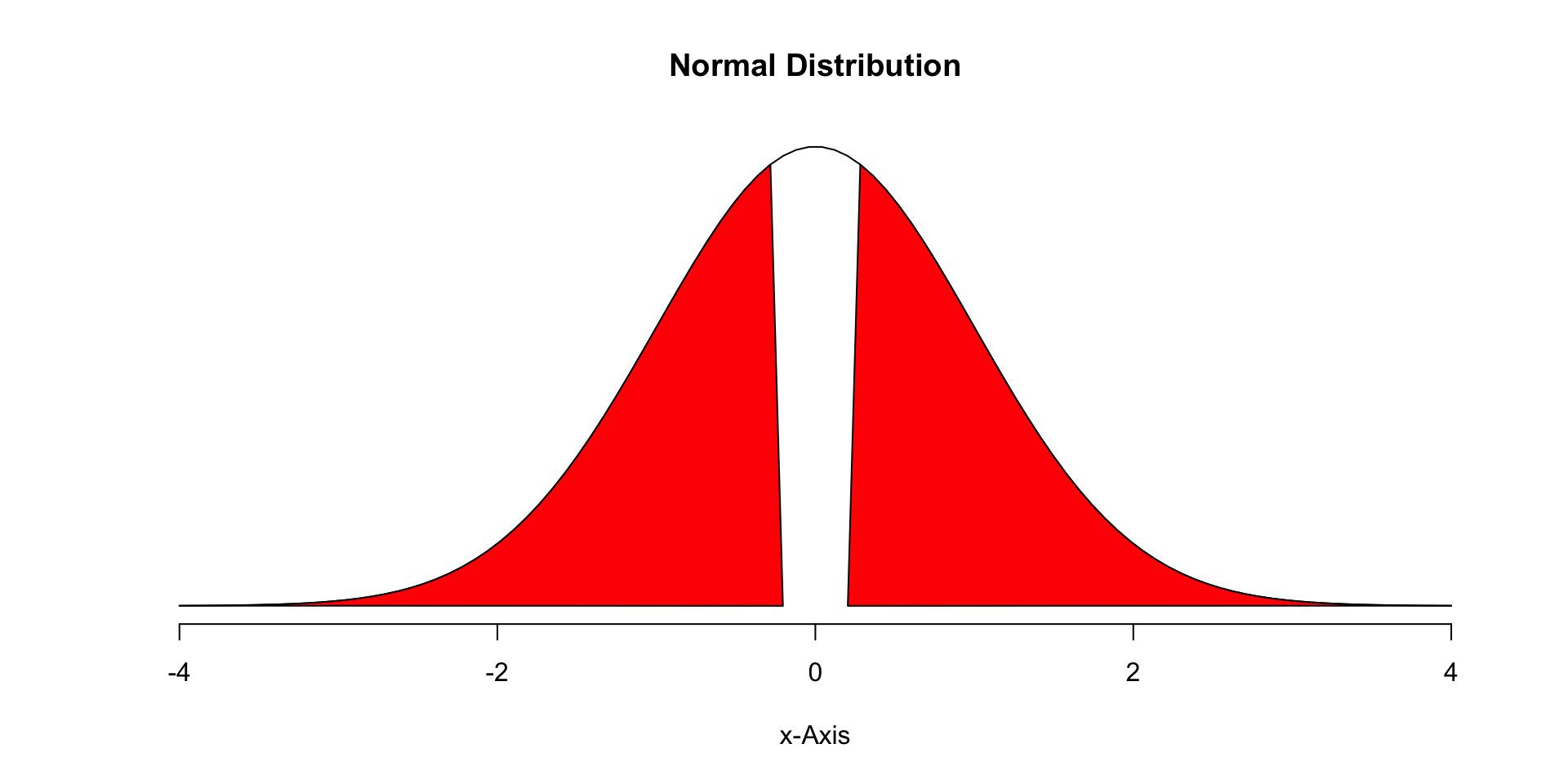March 6, 2019

## Announcements

• Data Project
• Proposal is due March 31st.
• Final project is May 16th.
• Information (including a template and example) is available here: http://data606.net/assignments/project/
• Important points about the proposal:
• Your data set should have three variables (two quantitative, one qualitative) such that you predict one quantitative variable from the other two.
• Include relavant descriptive statistics and at least one visualization per variable.

## Population Distribution (Uniform)

n <- 1e5
pop <- runif(n, 0, 1)
mean(pop)
##  0.5008915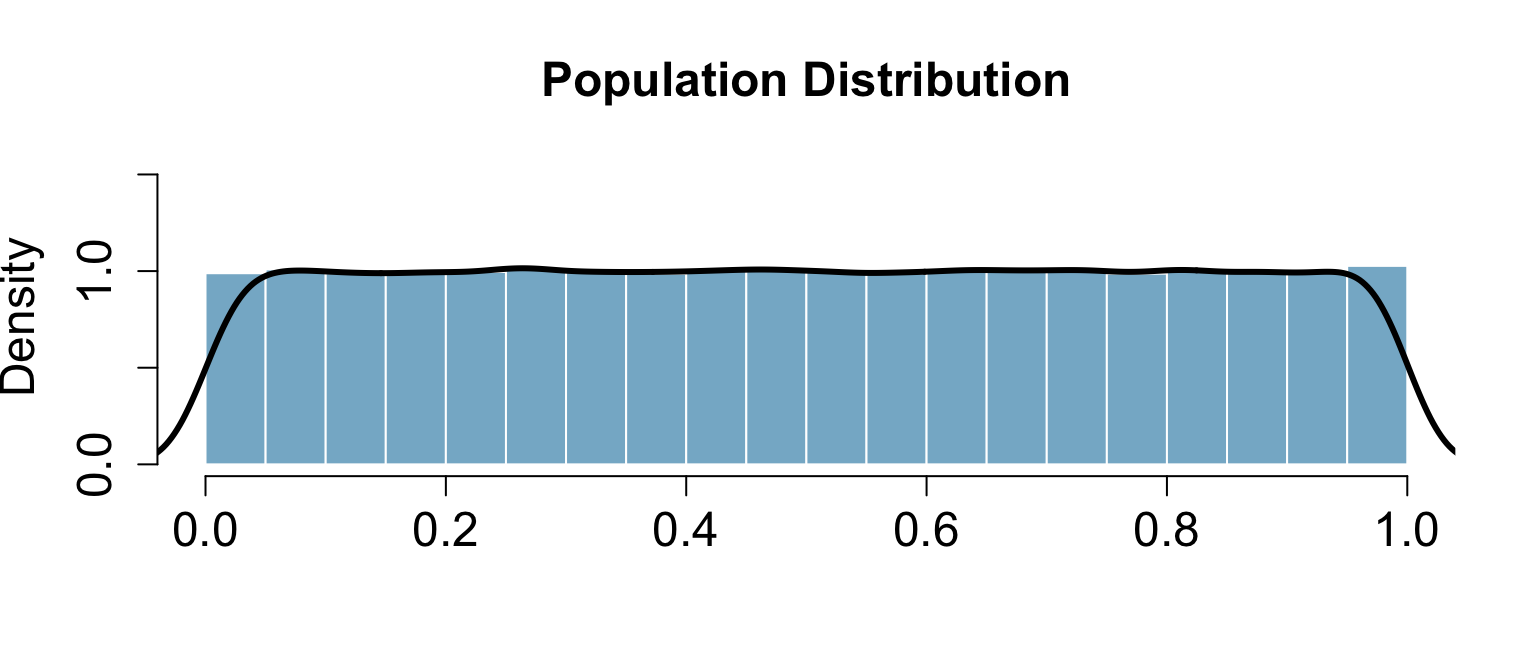## Random Sample (n=10)

samp1 <- sample(pop, size=10)
mean(samp1)
##  0.5745289
hist(samp1)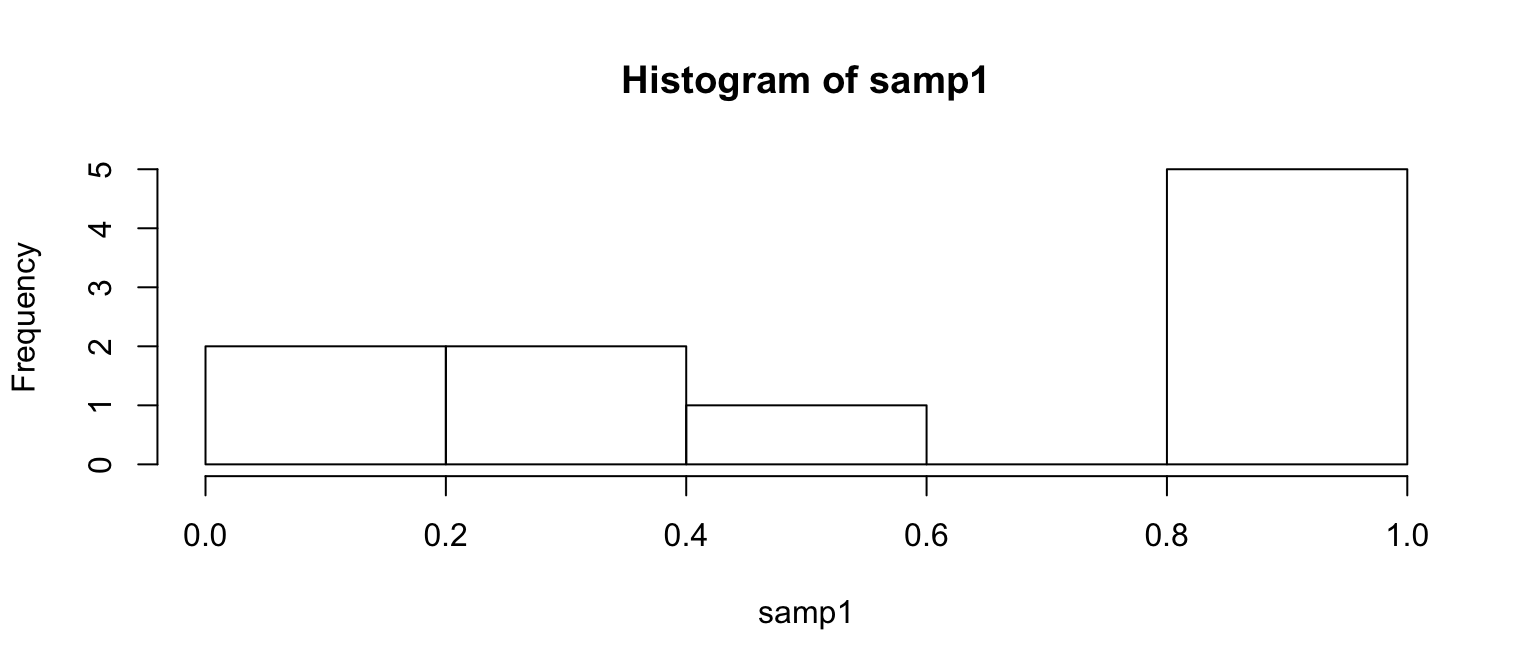## Random Sample (n=30)

samp2 <- sample(pop, size=30)
mean(samp2)
##  0.5466776
hist(samp2)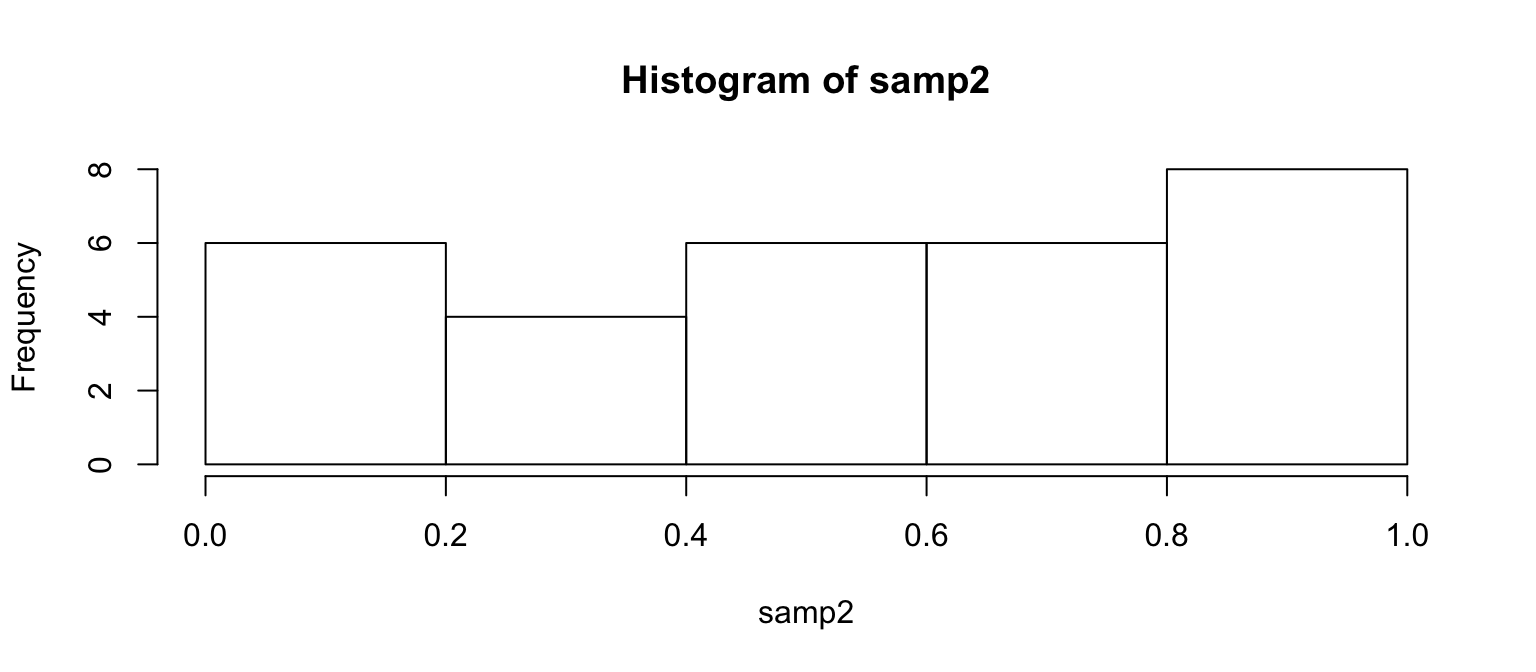## Lots of Random Samples

M <- 1000
samples <- numeric(length=M)
for(i in seq_len(M)) {
samples[i] <- mean(sample(pop, size=30))
}
head(samples, n=8)
##  0.5294721 0.4424369 0.5102434 0.4409382 0.5492505 0.5829651 0.5322821
##  0.5063398

## Sampling Distribution

hist(samples)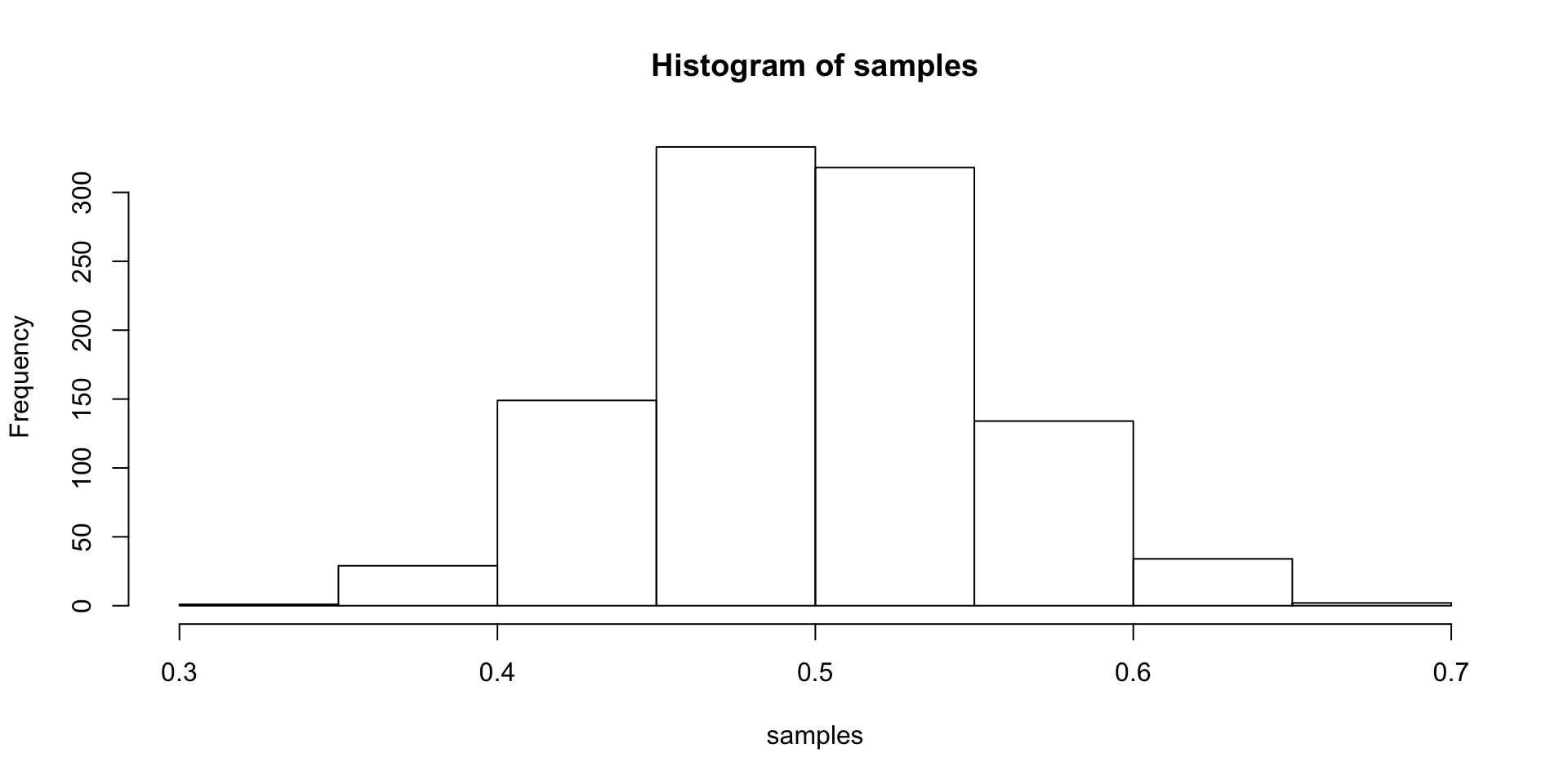## Central Limit Theorem (CLT)

Let $$X_1$$, $$X_2$$, …, $$X_n$$ be independent, identically distributed random variables with mean $$\mu$$ and variance $$\sigma^2$$, both finite. Then for any constant $$z$$,

$\underset { n\rightarrow \infty }{ lim } P\left( \frac { \bar { X } -\mu }{ \sigma /\sqrt { n } } \le z \right) =\Phi \left( z \right)$

where $$\Phi$$ is the cumulative distribution function (cdf) of the standard normal distribution.

## In other words…

The distribution of the sample mean is well approximated by a normal model:

$\bar { x } \sim N\left( mean=\mu ,SE=\frac { \sigma }{ \sqrt { n } } \right)$

where SE represents the standard error, which is defined as the standard deviation of the sampling distribution. In most cases $$\sigma$$ is not known, so use $$s$$.

## CLT Shiny App

shiny_demo('sampdist')
shiny_demo('CLT_mean')

## Standard Error and Confidence Interval

samp2 <- sample(pop, size=30)
mean(samp2)
##  0.5747245
(samp2.se <- sd(samp2) / sqrt(length(samp2)))
##  0.04941707

The confidence interval is then $$\mu \pm 2 \times SE$$

(samp2.ci <- c(mean(samp2) - 2 * samp2.se, mean(samp2) + 2 * samp2.se))
##  0.4758903 0.6735586

## Confidence Intervals

We are 95% confident that the true population mean is between 0.4758903, 0.6735586.

That is, if we were to take 100 random samples, we would expect at least 95% of those samples to have a mean within 0.4758903, 0.6735586.

ci <- data.frame(mean=numeric(), min=numeric(), max=numeric())
for(i in seq_len(100)) {
samp <- sample(pop, size=30)
se <- sd(samp) / sqrt(length(samp))
ci[i,] <- c(mean(samp),
mean(samp) - 2 * se,
mean(samp) + 2 * se)
}
ci$sample <- 1:nrow(ci) ci$sig <- ci$min < 0.5 & ci$max > 0.5

## Confidence Intervals

ggplot(ci, aes(x=min, xend=max, y=sample, yend=sample, color=sig)) +
geom_vline(xintercept=0.5) +
geom_segment() + xlab('CI') + ylab('') +
scale_color_manual(values=c('TRUE'='grey', 'FALSE'='red'))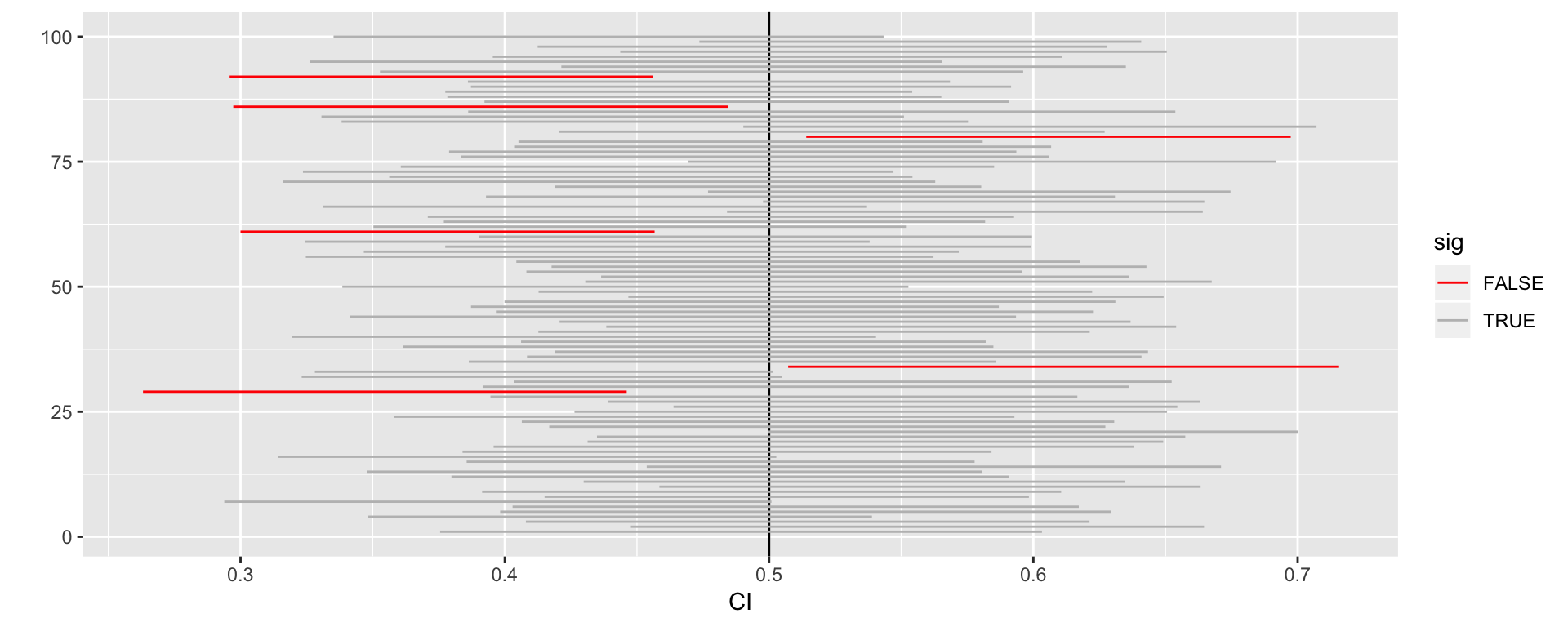## Hypothesis Testing

• We start with a null hypothesis ($$H_0$$) that represents the status quo.
• We also have an alternative hypothesis ($$H_A$$) that represents our research question, i.e. what we’re testing for.
• We conduct a hypothesis test under the assumption that the null hypothesis is true, either via simulation or traditional methods based on the central limit theorem.
• If the test results suggest that the data do not provide convincing evidence for the alternative hypothesis, we stick with the null hypothesis. If they do, then we reject the null hypothesis in favor of the alternative.

## Hypothesis Testing (using CI)

$$H_0$$: The mean of samp2 = 0.5
$$H_A$$: The mean of samp2 $$\ne$$ 0.5

Using confidence intervals, if the null value is within the confidence interval, then we fail to reject the null hypothesis.

(samp2.ci <- c(mean(samp2) - 2 * sd(samp2) / sqrt(length(samp2)),
mean(samp2) + 2 * sd(samp2) / sqrt(length(samp2))))
##  0.4758903 0.6735586

Since 0.5 fall within 0.4758903, 0.6735586, we fail to reject the null hypothesis.

## Hypothesis Testing (using p-values)

$\bar { x } \sim N\left( mean=0.49,SE=\frac { 0.27 }{ \sqrt { 30 } = 0.049 } \right)$

$Z=\frac { \bar { x } -null }{ SE } =\frac { 0.49-0.50 }{ 0.049 } = -.204081633$

pnorm(-.204) * 2
##  0.8383535

## Hypothesis Testing (using p-values)

normalPlot(bounds=c(-.204, .204), tails=TRUE)